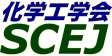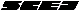## \$B9V1i%W%m%0%i%`!J%;%C%7%g%sJL!K(B

### \$B^5-G09V1i(B \$B!]2=3X9)3X2q5;=Q>^!](B

#### XE113-XE117

\$B:G=*99?7F|;~!'(B2012-03-09 14:23:08
\$B9V1i(B
\$B;~9o(B
\$B9V1i(B
\$BHV9f(B
\$B9V1iBjL\!?H/I=\$B%-!<%o!<%I(B\$BJ,N`(B
\$BHV9f(B
\$B\$BHV9f(B
XE\$B2q>l(B \$BBh(B1\$BF|(B
(13:00\$B!A(B13:20)\$B!!(B(\$B;J2q(B \$B@6?e(B \$BCiL@(B)
13:00\$B!A(B 13:20XE113[\$B5;=Q>^(B] \$BGQ4~J*%j%5%\$%/%k\$K8~\$1\$?4%<0Hf=EJ,N%5;=Q(B
(\$B2,;3Bg(B) \$B!{(B(\$B@5(B)\$B2!C+(B \$B=a(B \$B!&(B (\$B1JED%(%s%8%K%"%j%s%0(B) \$B5WJ](B \$BBYM:(B \$B!&(B \$B?^;U(B \$BC#Li(B \$B!&(B \$BCfL3(B \$B??8c(B
gijutusyou
0-c764
(13:20\$B!A(B13:40)\$B!!(B(\$B;J2q(B \$BETN1(B \$BL-N;(B)
13:20\$B!A(B 13:40XE114[\$B5;=Q>^(B] \$B9b=cEY%U%k%*%l%sM6F3BN\$N@=B\$5;=Q\$H%*%W%H%(%l%/%H%m%K%/%9:`NA\$H\$7\$F\$N1~MQ(B
(\$BBg:e%,%9(B) \$B!{@n:j(B \$B??0l(B \$B!&(B \$B5\Fb(B \$B?.Je(B \$B!&(B (\$BBg:e%,%9%1%_%+%k(B) \$B?\ED(B \$B9/M5(B \$B!&(B \$B;3ED(B \$B8w><(B \$B!&(B \$BB<@%(B \$BM5L@(B
gijutusyou
0-c765
(13:40\$B!A(B14:00)\$B!!(B(\$B;J2q(B \$B^
13:40\$B!A(B 14:00XE115[\$B5;=Q>^(B] \$B%W%i%s%H\$N@_Hw!&1?E>\$NE}9g%b%G%k\$N3+H/!&Ia5Z(B
(\$B%F%/%^%9(B) \$B!{2OB<(B \$B9,Fs(B \$B!&(B \$BCg(B \$BM&<#(B \$B!&(B \$BHSED(B \$B5.9,(B \$B!&(B \$BN?(B \$B856S(B \$B!&(B \$B
gijutusyou
0-c767
(14:00\$B!A(B14:20)\$B!!(B(\$B;J2q(B \$BEDC+(B \$B@5?N(B)
14:00\$B!A(B 14:20XE116[\$B5;=Q>^(B] \$B%0%j%3%j%I5Z\$S%]%j%0%j%3!<%k;@(B(PGA)\$B\$N9)6HE*@8;:%W%m%;%9\$N3+H/(B
(\$B%/%l%O(B) \$B;g3@(B \$BM3>k(B \$B!&(B \$BI4@%(B \$B9n(B \$B!&(B \$B!{BgED86(B \$B7rB@O:(B \$B!&(B (KUREHA PGA LLC) \$B@1(B \$BCR9-(B \$B!&(B \$B?@;REg(B \$B9n(B
gijutusyou
0-c768
(14:20\$B!A(B14:40)\$B!!(B(\$B;J2q(B \$BIpF#(B \$BL@FA(B)
14:20\$B!A(B 14:40XE117[\$B5;=Q>^(B] \$B2r@O5;=Q\$r3hMQ\$7\$?%^%\$%/%m%j%"%/%?%7%9%F%`\$K\$h\$k%W%m%;%92~A1(B
(\$BF|N)@=:n=j(B) \$B!{IY3_(B \$B@9E5(B \$B!&(B \$B@uLn(B \$BM32V;R(B \$B!&(B \$BJR;3(B \$B3(N\$9a(B \$B!&(B (\$BF|N)%W%i%s%H%F%/%N%m%8!<(B) \$B1sF#(B \$B4n=E(B \$B!&(B \$B5\K\(B \$BE/O:(B
gijutusyou
0-c769

\$B9V1i%W%m%0%i%`(B
\$B2=3X9)3X2q(B \$BBh(B77\$BG/2q(B(C) 2012 \$B8x1W
Most recent update: 2012-03-09 14:23:08
E-mail: inquiry-77awww3.scej.org
This page was generated byeasp 2.28; proghtml 2.28b (C)1999-2011 kawase